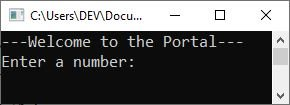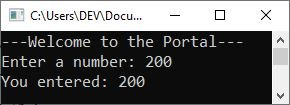Overloading occurs when the same function name is used in multiple function declarations within the same scope. If C++ can tell them apart based on their number and types of arguments, they can share the same name. In C++, for example, the following four functions are distinct:

```float divide(int a, int b);
float divide(int a, int b, int c);
float divide(float x, float y);
float divide(float x, float y, float z);```

That is, divide() taking two int type arguments is different from divide() taking three int type arguments, and divide() taking two float type arguments is different from divide() taking three float type arguments. This is known as "function overloading."

As an example of function overloading in C++, consider the following program.

```#include <iostream>
using namespace std;

void myFunction();
void myFunction(int);

int main()
{
myFunction();

int num;
cout<<"Enter a number: ";
cin>>num;

myFunction(num);

cout<<endl;
return 0;
}

void myFunction()
{
cout<<"---Welcome to the Portal---\n";
}
void myFunction(int x)
{
cout<<"You entered: "<<x;
}```

The following snapshot shows the initial output produced by the above example program:Now type a number, say 200, and hit the ENTER key to produce the following output:Now, let me briefly explain the preceding program to you:

• First, I declared the function prototype of "myFunction()," which does not return a value and does not accept a parameter.
• After that, I declared another function prototype with the same name, "myFunction()." As a result, you can say that I overloaded the function "myFunction()." But the second function allows one "int" parameter to be received or accepted.
• I called the function "myFunction()" within the "main()" method because I did not provide a parameter when calling, so the first "myFunction()" will be called, which prints the text "Welcome to the Portal," followed by a new line on the output console.
• Then, inside "main()," I received a number from the user during program execution, which I assigned to the "num" variable, and I passed this variable as a parameter to the function "myFunction()" a second time. As a result, the parameter function will be called, followed by the text "You entered:" and the value of "num," or the value entered by the user during program execution.

Before concluding the discussion of "function overloading in C++," allow me to provide one more example.

```#include <iostream>
using namespace std;
void myFunction();
void myFunction(int);
void myFunction(int, int);
void myFunction(int, float);
float myFunction(float);
int main()
{
int a = 2, b = 4;
float x = 2.4;
myFunction();
myFunction(a);
myFunction(a, b);
myFunction(a, x);
cout<<"Area of circle with radius "<<x<<" = "<<myFunction(x);
cout<<endl;
return 0;
}
void myFunction()
{
cout<<"Welcome to the Portal\n\n";
}
void myFunction(int val)
{
cout<<"The value of the parameter is: "<<val<<"\n\n";
}
void myFunction(int valOne, int valTwo)
{
cout<<valOne<<" + "<<valTwo<<" = "<<valOne+valTwo<<"\n\n";
}
void myFunction(int valOne, float valTwo)
{
cout<<valOne<<" + "<<valTwo<<" = "<<valOne+valTwo<<"\n\n";
}
float myFunction(float val)
{
return (3.14 * val * val);
}```

The output should be:

```Welcome to the Portal

The value of the parameter is: 2

2 + 4 = 6

2 + 2.4 = 4.4

Area of circle with radius 2.4 = 18.0864```

The program execution always starts with the "main()" function. As a result, two variables, "a" and "b," with values of "2" and "4", were defined within the "main()" function. And using the second statement, a variable of type "float" was defined with a value of "2.4." Now I called the function "myFunction()" using the following statement:

`myFunction();`

Since I have not provided any arguments to it, the function without arguments will be called, the first one that prints the text "Welcome to the Portal" on the output console. Now, using the following statement:

`myFunction(a);`

I called the same function but provided an integer parameter; thus, the function that accepts one "int" parameter, that is, the second function, which will print the text "The value of the parameter is: " followed by the value of the parameter passed to it, will be executed. Since I passed "a" as its parameter, whose value is "2," therefore, the following was the output produced:

`The value of the parameter is: 2`

I again called the function "myFunction()," but passed the two "int" parameters this time, using the following statement:

`myFunction(a, b);`

Therefore, the following code will be executed: This code is written as the function definition of the function "myFunction()," whose return type is "void," and which accepts two "int" parameters.

`cout<<valOne<<" + "<<valTwo<<" = "<<valOne+valTwo<<"\n\n";`

Now I called the same function a fourth time using the following statement:

`myFunction(a, x);`

This time, I supplied two parameters, one of "int" type and the other of "float" type; thus, "myFunction()," which accepts two parameters, one of "int" type and the other of "float" type, will be called and executed. The output will be the same as that of the previous statement; the only difference is that because the second passed argument was "x," whose value is "2.4," the output should be:

`2 + 2.4 = 4.4`

Finally, using the following statement:

`cout<<"Area of circle with radius "<<x<<" = "<<myFunction(x);`

The text "Area of a circle with a radius of 2.4" is printed, and the function "myFunction()" is called. Since I provided one parameter of the "float" type, therefore, the last "myFunction()" function was called and executed, which will find the area of the circle using the passed parameter as the radius of the circle. Therefore, since the value of "x" is "2.4," the output should be:

`Area of circle with radius 2.4 = 18.0864`

18.0864 is the result of "3.14 * 2.4 * 2.4," the value returned by the function.

C++ Quiz

« Previous Tutorial Next Tutorial »